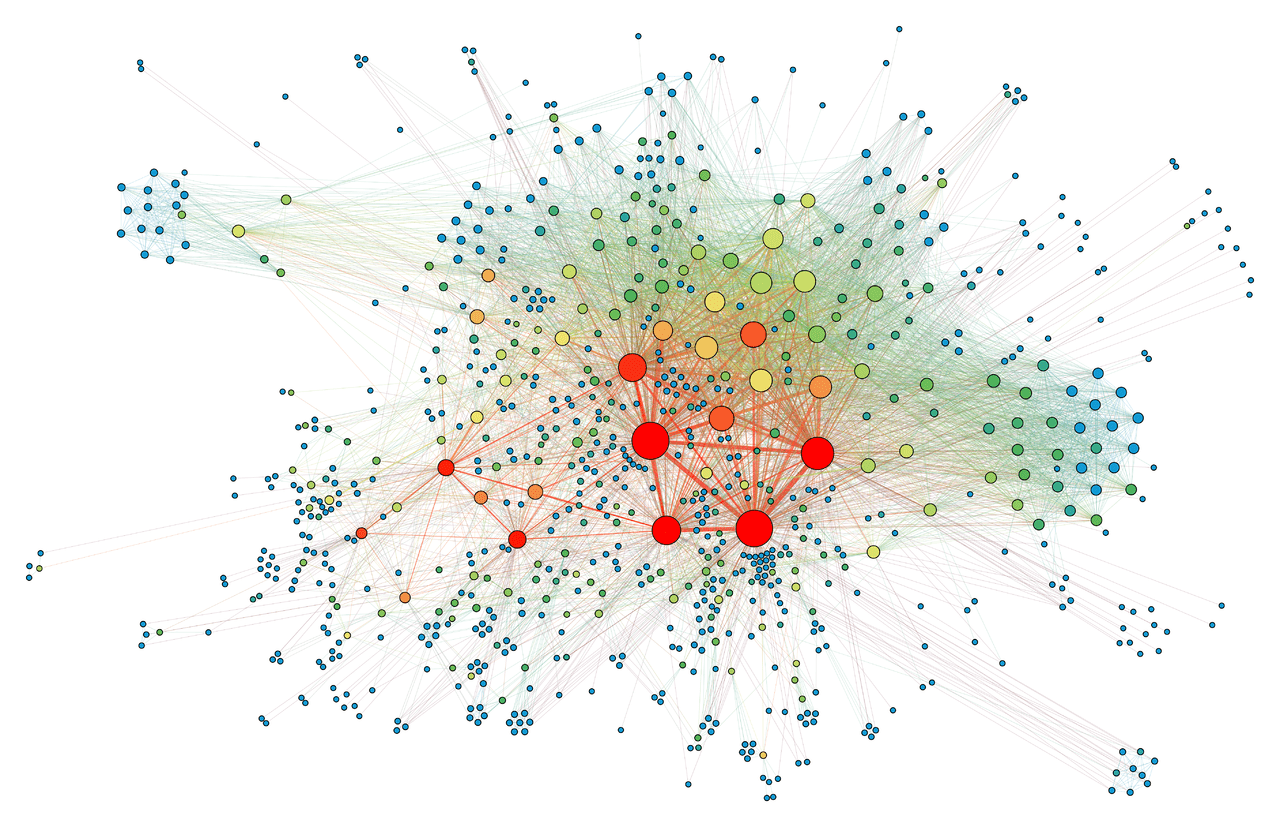# Force Directed Graph - What is it ?

A Force-Directed Graph, or Force-Based Graph, is a type of layout commonly used in a variety of application areas: network visualization, large graph visualization, knowledge representation, system management, or mesh visualization.

It is used to visualize the connections between objects in a network. By grouping the objects connected to each other in a natural way, a Force-Directed Graph is visually interesting and also makes it possible to discover subtle relationships between groups.

These graphs have the particularity of being drawn by algorithms called "force-directed graph drawing algorithms" or "force-directed graph drawing algorithms".

They are also sometimes referred to as "spring-embedder" algorithms or "energy-based placement" algorithms. This algorithm arranges the graphs in a natural and aesthetically pleasing way, usually resulting in a symmetrical and clustered structure. The distribution of the different "nodes" is harmonious and well balanced.

The algorithm is based on a physical model. It calculates the layout of the graph based solely on the information contained in the graph structure rather than relying on domain-specific knowledge.

The nodes are represented by points that repel each other like magnets. Borders connect these points by simulating a spring force to attract adjacent nodes.

The model determines the forces acting on the nodes iteratively and moves them in order to come as close as possible to an equilibrium where the position of the nodes remains stable.

Tutte's algorithm, created in 1963, is one of the first graphing methods based on barycentric representations. Previously, the Eades layout method and the Frucherman and Rein-gold algorithm were already based on spring forces similar to those of Hooke's law.

The forces between nodes can also be calculated based on their theoretical distances, determined by the length of the shortest path between them. Kamada and Kawai's algorithm uses spring forces proportional to the theoretical distances in the graph.The great advantage of this type of Force-Directed graph plotting algorithm is the simplicity of its implementation. However, it works best for graphs where the number of lines is similar to the number of points. Denser graphs with many lines or unstructured graphs are less suitable.

If the directions of the lines are too large, this is also not the best choice. The reason for this is that this visualization method does not take these directions into account.